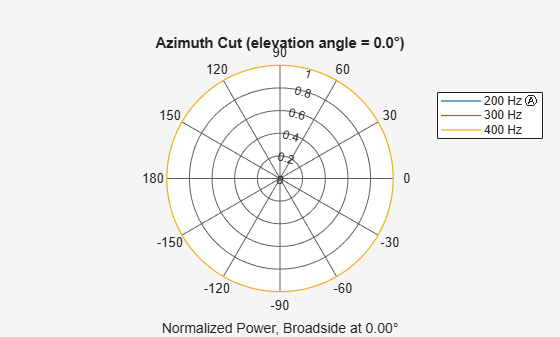# step

System object: phased.OmnidirectionalMicrophoneElement
Package: phased

Output response of microphone

## Syntax

```RESP = step(H,FREQ,ANG) ```

## Description

Note

Starting in R2016b, instead of using the `step` method to perform the operation defined by the System object™, you can call the object with arguments, as if it were a function. For example, ```y = step(obj,x)``` and `y = obj(x)` perform equivalent operations.

`RESP = step(H,FREQ,ANG)` returns the microphone’s magnitude response, `RESP`, at frequencies specified in `FREQ` and directions specified in `ANG`.

Note

The object performs an initialization the first time the object is executed. This initialization locks nontunable properties and input specifications, such as dimensions, complexity, and data type of the input data. If you change a nontunable property or an input specification, the System object issues an error. To change nontunable properties or inputs, you must first call the `release` method to unlock the object.

## Input Arguments

 `H` Microphone object. `FREQ` Frequencies in hertz. `FREQ` is a row vector of length L. `ANG` Directions in degrees. `ANG` can be either a 2-by-M matrix or a row vector of length M. If `ANG` is a 2-by-M matrix, each column of the matrix specifies the direction in the form [azimuth; elevation]. The azimuth angle must be between –180 and 180 degrees, inclusive. The elevation angle must be between –90 and 90 degrees, inclusive. If `ANG` is a row vector of length M, each element specifies a direction’s azimuth angle. In this case, the corresponding elevation angle is assumed to be 0.

## Output Arguments

 `RESP` Response of microphone. `RESP` is an M-by-L matrix that contains the responses of the microphone element at the M angles specified in `ANG` and the L frequencies specified in `FREQ`.

## Examples

expand all

Create an omnidirectional microphone. Find the microphone response at 200, 300, and 400 Hz for the incident angle 0° azimuth and 0° elevation. Then, plot the azimuth response of the microphone at three frequencies.

```microphone = phased.OmnidirectionalMicrophoneElement(... 'FrequencyRange',[20 2e3]); fc = [200 300 400]; ang = [0;0]; resp = microphone(fc,ang);```

Plot the response pattern. Response patterns for all three frequencies are the same.

`pattern(microphone,fc,[-180:180],0,'CoordinateSystem','polar','Type','power');`